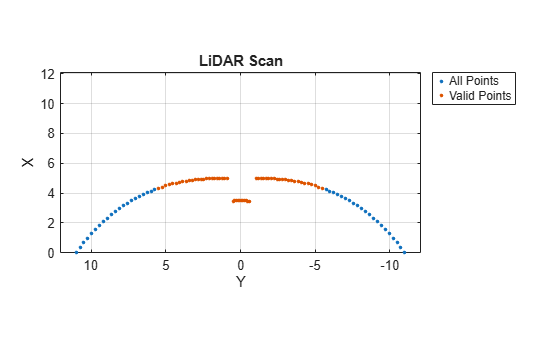# removeInvalidData

Remove invalid range and angle data

## Syntax

``validScan = removeInvalidData(scan)``
``validScan = removeInvalidData(scan,Name,Value)``

## Description

````validScan = removeInvalidData(scan)`returns a new `lidarScan` object with all `Inf` and `NaN` values from the input `scan` removed. The corresponding angle readings are also removed.```

example

````validScan = removeInvalidData(scan,Name,Value)`provides additional options specified by one or more `Name,Value` pairs.```

## Examples

collapse all

Specify lidar data as vectors of ranges and angles. These values include readings outside of the sensors range.

```x = linspace(-2,2); ranges = abs((1.5).*x.^2 + 5); ranges(45:55) = 3.5; angles = linspace(-pi/2,pi/2,numel(ranges));```

Create a lidar scan by specifying the ranges and angles. Plot all points of the lidar scan.

```scan = lidarScan(ranges,angles); plot(scan)```Remove invalid points based on a specified minimum and maximum range.

```minRange = 0.1; maxRange = 7; scan2 = removeInvalidData(scan,'RangeLimits',[minRange maxRange]); hold on plot(scan2) legend('All Points','Valid Points')```## Input Arguments

collapse all

Lidar scan readings, specified as a `lidarScan` object.

### Name-Value Arguments

Specify optional pairs of arguments as `Name1=Value1,...,NameN=ValueN`, where `Name` is the argument name and `Value` is the corresponding value. Name-value arguments must appear after other arguments, but the order of the pairs does not matter.

Before R2021a, use commas to separate each name and value, and enclose `Name` in quotes.

Example: `["RangeLimits",[0.05 2]`

Range reading limits, specified as a two-element vector, `[minRange maxRange]`, in meters. All range readings and corresponding angles outside these range limits are removed

Data Types: `single` | `double`

Angle limits, specified as a two-element vector, ```[minAngle maxAngle]``` in radians. All angles and corresponding range readings outside these angle limits are removed.

Angles are measured counter-clockwise around the positivez-axis.

Data Types: `single` | `double`

## Output Arguments

collapse all

Lidar scan readings, specified as a `lidarScan` object. All invalid lidar scan readings are removed.

## Version History

Introduced in R2017b